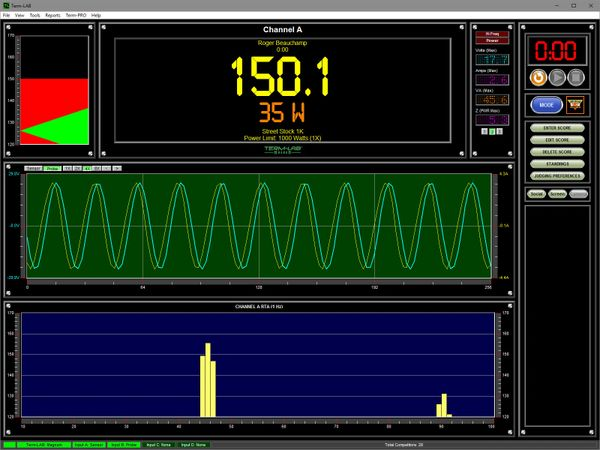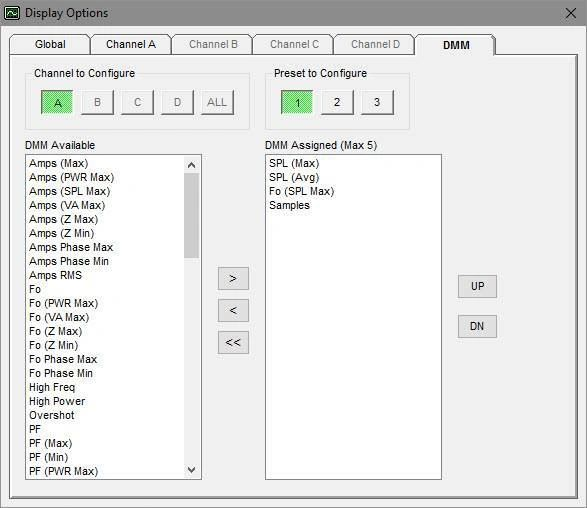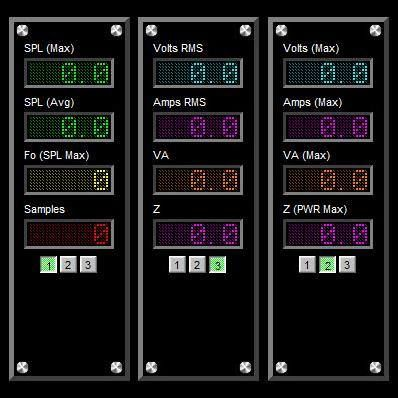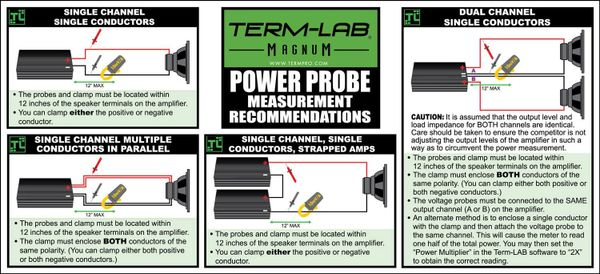•••••• # Term-LAB Digital Power Probe Kit

\$269.00

The Term-LAB Magnum Precision Power Probe peripheral adds amplifier power measurement capability to your Term-LAB Magnum or Rebel (not included). Adds more than 70 additional measurements to your Term-LAB Magnum or Rebel.

>> IN STOCK - Most Orders ship same day via FedEx<<

• Real-Time True RMS Power Measurements
• 200 Vrms Maximum Input Voltage
• 400 Amps Maximum AC Current
• Measures Single Ended or Bridged Amplifier Output
 Included Components
• Precision Power Probe Interface
• Inductive AC Current Clamp
 Screen ShotsTrue power readings are displayed on the primary readout (orange). The current and voltage waveforms may displayed on the scope. User selectable power related measurements can be assigned to the smaller DMM readouts to the right of the primary display.The Term-LAB Power Probe adds more than 70 additional measurements to the Term-LAB Magnum or Rebel. The user can assign these measurements to the various DMM readouts.Each channel in Term-LAB has three banks of DMM readouts. Each bank can display up to 5 DMM readouts. Each readout can be individually assigned by the user. = Complete list of DMM (Digital Multi Meter) Measurements = • Amps (Max) - Maximum AC speaker current in amps (rms)• Amps (PWR Max) - AC speaker current in amps (rms) at maximum true power• Amps (SPL Max) - AC speaker current in amps (rms) at maximum SPL• Amps (VA Max) - AC speaker current in amps (rms) at VA maximum• Amps (Z Max) - AC speaker current in amps (rms) at maximum calculated load impedance Z• Amps (Z Min) - AC speaker current in amps (rms) at minimum calculated load impedance Z• Amps Phase Max - AC speaker current in amps (rms) at the maximum V/A phase shift• Amps Phase Min - AC speaker current in amps (rms) at the minimum V/A phase shift• Amps RMS - Real time AC speaker current in amps (rms)• Fo• Fo (PWR Max) - Frequency in Hz at maximum true power• Fo (SPL Max) - Frequency in Hz at maximum SPL• Fo (VA Max) - Frequency in Hz at VA maximum• Fo (Z Max) - Frequency in Hz at maximum calculated load impedance Z• Fo (Z Min) - Frequency in Hz at minimum calculated load impedance Z• Fo Phase Max - Frequency in Hz at the maximum V/A phase shift• Fo Phase Min - Frequency in Hz at the minimum V/A phase shift• High Freq - High frequency violation flag• High Power - High power violation flag• Overshot - Overshot violation flag• PF - Real time calculated Power Factor• PF (Max) - Maximum calculated Power Factor• PF (Min) - Minimum calculated Power Factor• PF (PWR Max) - Calculated Power Factor at maximum true power• PF (SPL Max) - Calculated Power Factor at maximum SPL• PF (VA Max) - Calculated Power Factor at VA maximum• PF (Z Max) - Calculated Power Factor at maximum calculated load impedance Z• PF (Z Min) - Calculated Power Factor at minimum calculated load impedance Z• PF Phase Max - Calculated Power Factor at the maximum V/A phase shift• PF Phase Min - Calculated Power Factor at the minimum V/A phase shift• Phase - Real time calculated V/A Phase Shift in degrees• Phase (Max) - Maximum calculated V/A Phase Shift in degrees• Phase (Min) - Minimum calculated V/A Phase Shift in degrees• Phase (PWR Max) - Calculated V/A Phase Shift in degrees at maximum true power• Phase (SPL Max) - Calculated V/A Phase Shift in degrees at maximum SPL• Phase (VA Max) - Calculated V/A Phase Shift in degrees at VA maximum• Phase (Z Max) - Calculated V/A Phase Shift in degrees at maximum calculated load impedance Z• Phase (Z Min) - Calculated V/A Phase Shift in degrees at minimum calculated load impedance Z• Power (Max) - Maximum true power in Watts• Power (SPL Max) - True power in Watts at maximum SPL• Power (True) - Real time true power in watts• Power (VA Max) - True power in watts at VA maximum• Power (Z Max) - True power in watts at maximum calculated load impedance Z• Power (Z Min) - True power in watts at minimum calculated load impedance Z• Power Phase Max - True power in watts at the maximum V/A phase shift• Power Phase Min - True power in watts at the minimum V/A phase shift• Red Light - Red light violation flag• Samples - Number of samples taken since reset• SPL - Real time Sound Pressure Level in dB• SPL (Avg) - Average Sound Pressure Level in dB• SPL (Max) - Maximum Sound Pressure Level in dB• SPL (PWR Max) - Sound Pressure Level in dB at maximum true power• SPL (VA Max) - Sound Pressure Level in dB at VA maximum• SPL (Z Max) - Sound Pressure Level in dB at maximum calculated load impedance Z• SPL (Z Min) - Sound Pressure Level in dB at minimum calculated load impedance Z• SPL Phase Max - Sound Pressure Level in dB at the maximum V/A phase shift• SPL Phase Min - Sound Pressure Level in dB at the minimum V/A phase shift• VA - Volt Amps in Watts• VA (Max) - Maximum Volt Amps in Watts• VA (PWR Max) - Volt Amps in Watts at maximum true power• VA (SPL Max) - Volt Amps in Watts at maximum SPL• VA (Z Max) - Volt Amps in Watts at maximum calculated load impedance Z• VA (Z Min) - Volt Amps in Watts at minimum calculated load impedance Z• VA Phase Max - Volt Amps in Watts at the maximum V/A phase shift• VA Phase Min - Volt Amps in Watts at the minimum V/A phase shift• Volts (Max) - Maximum AC speaker voltage in volts (rms)• Volts (PWR Max) - AC speaker voltage in volts (rms) at maximum true power• Volts (SPL Max) - AC speaker voltage in volts (rms) at maximum SPL• Volts (VA Max) - AC speaker voltage in volts (rms) at VA maximum• Volts (Z Max) - AC speaker voltage in volts (rms) at maximum calculated load impedance Z• Volts (Z Min) - AC speaker voltage in volts (rms) at minimum calculated load impedance Z• Volts Phase Max - AC speaker voltage in volts (rms) at the maximum V/A phase shift• Volts Phase Min - AC speaker voltage in volts (rms) at the minimum V/A phase shift• Volts RMS - Real time AC speaker voltage in volts (rms)• Z - Real time calculated impedance in Ohms• Z (Max) - Maximum calculated impedance in Ohms• Z (Min) - Minimum calculated impedance in Ohms• Z (PWR Max) - Calculated impedance in Ohms at maximum true power• Z (SPL Max) - Calculated impedance in Ohms at maximum SPL• Z (VA Max) - Calculated impedance in Ohms at VA maximum• Z Phase Max - Calculated impedance in Ohms at the maximum V/A phase shift• Z Phase Min - Calculated impedance in Ohms at the minimum V/A phase shift